This post provides a less maths, more intuition’ overview of Analogies Explained: Towards Understanding Word Embeddings (ICML, 2019, Best Paper Honourable Mention). The outline follows that of the conference presentation. Target audience: general machine learning, NLP, computational linguistics.

## Background

### Word Embeddings

Word embeddings are numerical vector representations of words. Each entry, or dimension, of a word embedding can be thought of as capturing some semantic or syntactic feature of the word, and the full vector can be considered as co-ordinates of the word in a high-dimensional space. Word embeddings can be generated explicitly, e.g. from rows of word co-occurrence statistics (or low-rank approximations of such statistics); or by neural network methods such as Word2Vec (W2V) or Glove.

The latter, neural word embeddings, are found to be highly useful in natural language processing (NLP) tasks, such as evaluating word similarity, identifying named entities and assessing positive or negative sentiment in a passage of text (e.g. a customer review).

### Analogies

An intriguing property of neural word embeddings is that analogies can often be solved simply by adding/subtracting word embeddings. For example, the classic analogy:

man is to king as woman is to queen

can be solved using word embeddings by finding that closest to $\mathbf{w}_{king}-\mathbf{w}_{man}+\mathbf{w}_{woman}$, which turns out to be $\mathbf{w}_{queen}$ ($\mathbf{w}_{x}$ denotes the embedding of word $x$). Note that words in the question (e.g. $man$, $woman$ and $king$) are typically omitted from the search. This suggests the relationship:

$\begin{equation} \mathbf{w}_{king} - \mathbf{w}_{man} + \mathbf{w}_{woman} \approx \mathbf{w}_{queen}\, \tag{1}\label{eq:one} \end{equation}$

or, in geometric terms, that word embeddings of analogies approximately form parallelograms:.

Whilst this fits our intuition, the phenomenon is intriguing since word embeddings are not trained to achieve it! In practice, word embeddings of analogies do not perfectly form parallelograms: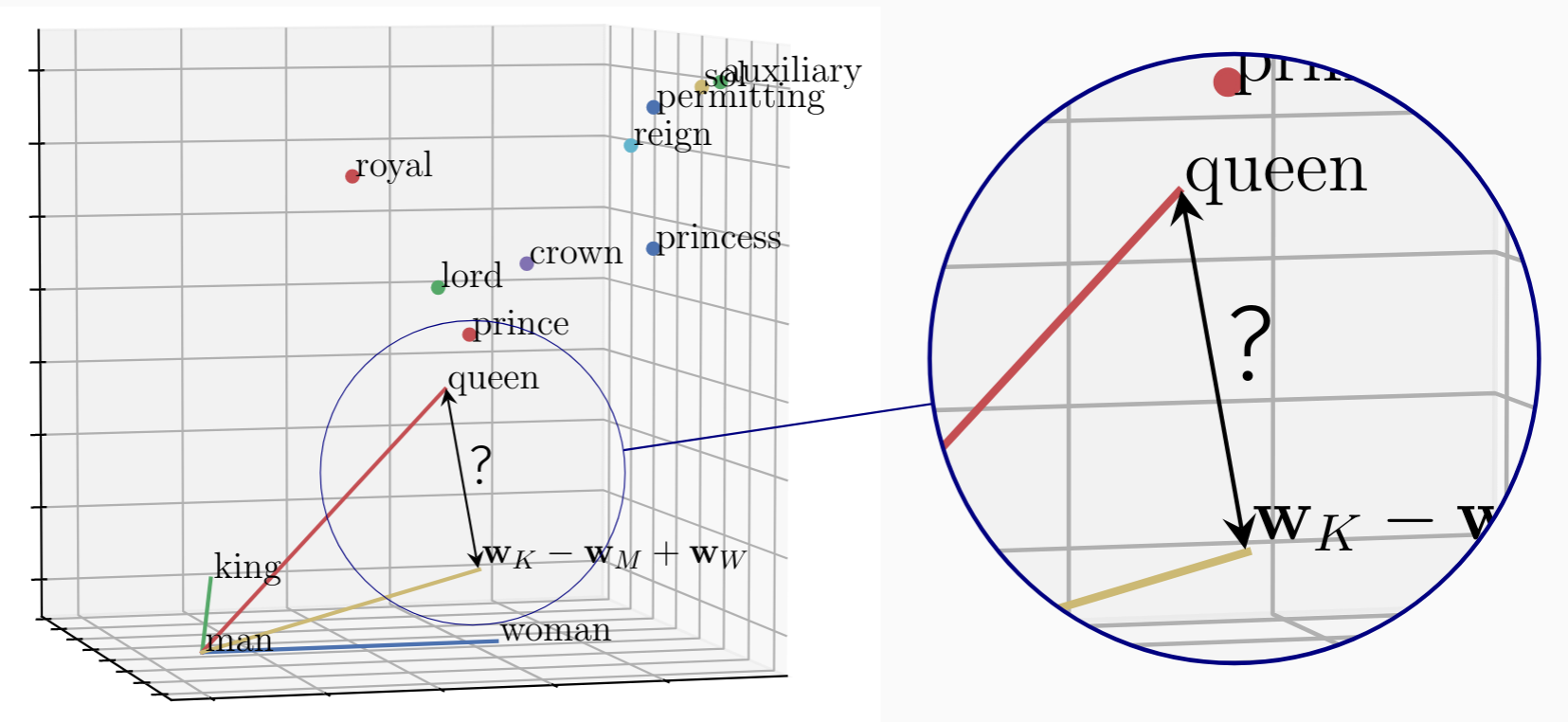This shows the (exact) parallelogram formed by $\mathbf{w}_{king}, \mathbf{w}_{man}$, $\mathbf{w}_{woman}$ and $\mathbf{w}_{king} {\small-} \mathbf{w}_{man} {\small+} \mathbf{w}_{woman}$ fixed in the $xy$-plane and a selection of word embeddings shown relative to it. We see that the embedding of $queen$ does not sit at its corner, but is the closest to it. Word embeddings of related words, e.g. $prince$ and $lord$, lie relatively close by and random unrelated words are further away.

 We explain the relationship between word embeddings of analogies \eqref{eq:one}, by explaining the gap (indicated) between $\mathbf{w}_{queen}$ and $\mathbf{w}_{king} {\small-} \mathbf{w}_{man} {\small+} \mathbf{w}_{woman}$; and why it is small, often smallest, for the word that completes the analogy.

To understand why semantic relationships between words give rise to geometric relationships between word embeddings, we first consider what W2V embeddings learn.

### Word2Vec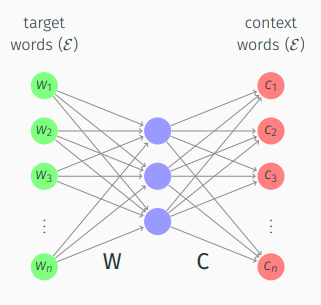W2V (SkipGram with negative sampling) is an algorithm that generates word embeddings by training the weights of a 2-layer “neural network” to predict context words $c_j$ (i.e. words that fall within a context window of fixed size $l$) around each word $w_i$ (referred to as a target word) across a text corpus.

Predicting $p(c_j\!\mid\! w_i)$, for all $c_j$ in a dictionary of all unique words $\mathcal{E}$, was initially considered with a softmax function, but instead a sigmoid function and negative sampling were used to reduce computational cost.

Levy & Goldberg (2014) showed that, as a result, the weight matrices $\mathbf{W}, \mathbf{C}$ (columns of which are the word embeddings $\mathbf{w}_i, \mathbf{c}_j$) approximately factorise a matrix of shifted Pointwise Mutual Information (PMI):

$\begin{equation} \mathbf{W}^\top\mathbf{C} \approx \textbf{PMI} - \log k\ , \end{equation}$

where $k$ is the chosen number of negative samples and $\begin{equation} \textbf{PMI}_{i,j} = \text{PMI}(w_i, c_j) = \log\tfrac{p(w_i,\, c_j)}{p(w_i)p(c_j)} \end{equation}\,.$

Dropping the shift term “$\log k$”, an artefact of the W2V algorithm (that we reconsider in the paper, Sec 5.5, 6.8), the relationship $\begin{equation} \mathbf{W}^\top\!\mathbf{C} \!\approx\! \textbf{PMI} \end{equation}$ shows that an embedding $\mathbf{w}_i$ can be considered a low-dimensional projection of a row of the PMI matrix, $\text{PMI}_i$ (a PMI vector).

## Proving the embedding relationship of analogies

From above, it can be seen that the additive relationship between word embeddings of analogies \eqref{eq:one} follows if (a) an equivalent relationship exists between PMI vectors, i.e.

$\begin{equation} \text{PMI}_{king} - \text{PMI}_{man} + \text{PMI}_{woman} \approx \text{PMI}_{queen}\ ; \tag{2}\label{eq:two} \end{equation}$

and (b) vector addition is sufficiently preserved under the low-rank projection induced by the loss function, as readily achieved by a least squares loss function and as approximated by W2V and Glove.

 To prove that relationship \eqref{eq:two} arises between PMI vectors of an analogy, we show that \eqref{eq:two} follows from a particular paraphrase relationship, which is, in turn, shown to be equivalent to an analogy.

### Paraphrases

When we say a word $w_*$ paraphrases a set of words $\mathcal{W}$, we mean, intuitively, that they are semantically interchangeable in the text. For example, where $king$ appears, we might instead see $man$ and $royal$ close together. Mathematically, a best choice paraphrase word $w_*$ can be defined as that which maximises the likelihood of the context words observed around $\mathcal{W}$. In other words, the distribution of words observed around $w_*$ defined over the dictionary $p(\mathcal{E}\!\mid\!w_*)$, should be similar to that around $\mathcal{W}$ $p(\mathcal{E}\!\mid\!\mathcal{W})$, as measured by Kullback-Leibler (KL) divergence. Whilst these distributions are discrete and unordered, we can picture this as: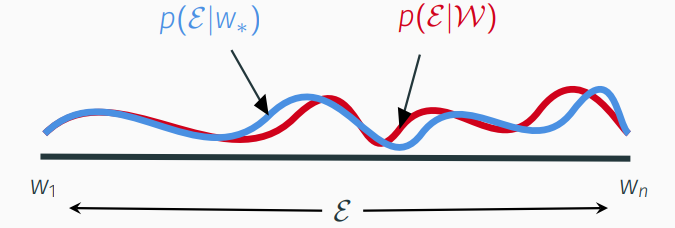Formally, we say $w_*$ paraphrases $\mathcal{W}$ if the paraphrase error ${\rho}^{\mathcal{W}, w_*}\!\in\! \mathbb{R}^{n}$ is (element-wise) small, where:

$\begin{equation} {\rho}^{\mathcal{W}, w_*}_j = \log \tfrac{p(c_j|w_*)}{p(c_j|\mathcal{W})}\ , \quad c_j\!\in\!\mathcal{E}. \end{equation}$

To see the relevance of paraphrases, we compare the sum of PMI vectors of two words $w_1, w_2$ to that of any word $w_*$ by considering each ($j^{th}$) component of the difference vector $\text{PMI}_* - (\text{PMI}_1 + \text{PMI}_2)$: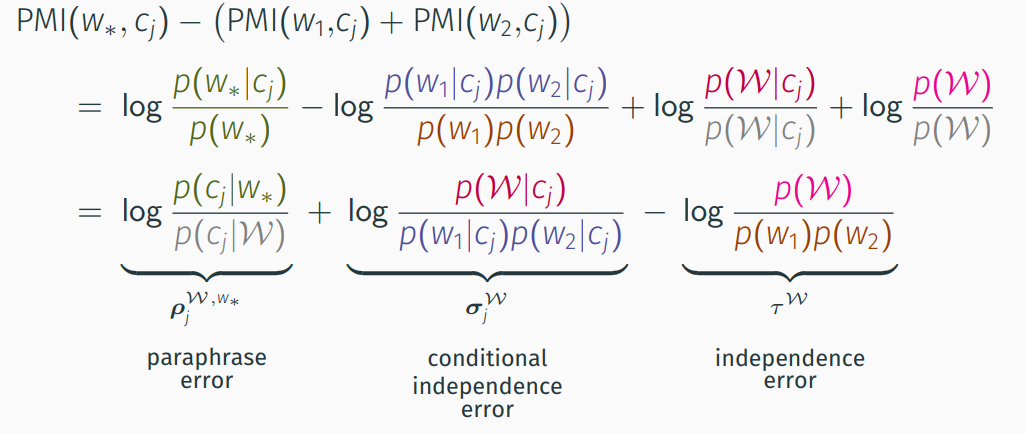We see that the difference can be written as a paraphrase error, small only if $w_*$ paraphrases $\{w_1, w_2\}$, and dependence error terms inherent to $w_1$ and $w_2$ that do not depend on $w_*$. Formally, we have:

Lemma 1: For any word $w_*\!\in\!\mathcal{E}$ and word set $\mathcal{W}\!\subseteq\!\mathcal{E}$, $|\mathcal{W}|\!<\!l$, where $l$ is the context window size:

$\begin{equation} \text{PMI}_* \ =\ \sum_{w_i\in\mathcal{W}} \text{PMI}_{i} \,+\, \rho^{\mathcal{W}, w_*} \,+\, \sigma^{\mathcal{W}} \,-\, \tau^\mathcal{W}\mathbf{1}\ . \end{equation}$

This connects paraphrasing to PMI vector addition, as appears in \eqref{eq:two}. To develop this further, paraphrasing is generalised, replacing $w_*$ by $\mathcal{W}_*$, to a relationship between any two word sets. The underlying principle remains the same: word sets paraphrase one another if the distributions of context words around them are similar (indeed, the original paraphrase definition is recovered if $\mathcal{W}_*$ contains a single word). Analogously to above, we find:

Lemma 2: For any word sets $\mathcal{W}, \mathcal{W}_*\!\subseteq\!\mathcal{E}$; $|\mathcal{W}|, |\mathcal{W}_*|\!<\!l$:

$\begin{equation} \sum_{w_i\in\mathcal{W}_*} \text{PMI}_{i} \ =\ \sum_{w_i\in\mathcal{W}} \text{PMI}_{i} \,+\, \rho^{\mathcal{W}, \mathcal{W}_*} \,+\, (\sigma^{\mathcal{W}} - \sigma^{\mathcal{W}_*}) \,-\, (\tau^\mathcal{W} - \tau^{\mathcal{W}_*})\mathbf{1} \end{equation}$

We can apply this to our example by setting $\mathcal{W} \!=\! \{woman, king\}$ and $\mathcal{W}_* \!=\! \{man, queen\}$, whereby

$\begin{equation} \text{PMI}_{king} - \text{PMI}_{man} + \text{PMI}_{woman} \ \approx\ \text{PMI}_{queen}\ , \end{equation}$

distributions](/assets/analogy/eqn_paraphrase.png){:height=”115px”} {: style=”text-align: left; margin-left: 30px”})

if $\mathcal{W}$ paraphrases $\mathcal{W}_*$ (meaning $\rho^{\mathcal{W}, \mathcal{W}_*}$ is small), subject to net statistical dependencies of words within $\mathcal{W}$ and $\mathcal{W}_*$, i.e. $\sigma^{\mathcal{W}} - \sigma^{\mathcal{W}_*}$ and $\tau^\mathcal{W} - \tau^{\mathcal{W}_*}$.

 Thus, \eqref{eq:two} holds, subject to dependence error, if $\{woman, king\}$ paraphrases $\{man, queen\}$.

This establishes a semantic relationship as a sufficient condition for the geometric relationship we aim to explain – but that semantic relationship is not an analogy. What remains then, is to explain why a general analogy “$w_a\text{ is to }w_{a^*}\text{ as }w_b\text{ is to }w_{b^*}$” implies that $\{w_b, w_{a^*}\!\}$ paraphrases $\{w_a, w_{b^*}\!\}$. We show that these conditions are in fact equivalent by reinterpreting paraphrases as word transformations.

### Word Transformation

From above, the paraphrase of a word set $\mathcal{W}$ by a word $w_*$ can be thought of as drawing a semantic equivalence between $\mathcal{W}$ and $w_*$. Alternatively, we can choose a particular word $w$ in $\mathcal{W}$ and view the paraphrase as indicating words (i.e. all others in $\mathcal{W}$, denoted $\mathcal{W}^+$) that, when added to $w$, make it “more like” – or transform it to – $w_*$. For example, the paraphrase of $\{man, royal\}$ by $king$ can be interpreted as a word transformation from $man$ to $king$ by adding $royal$. In effect, the added words narrow the context. More precisely, they alter the distribution of context words found around $w$ to more closely align with that of $w_*$. Denoting a paraphrase by $\approx_p$, we can represent this as: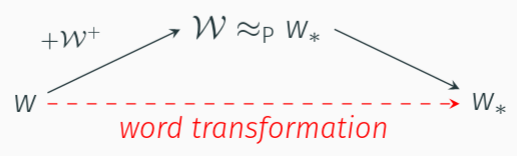in which the paraphrase can be seen as the “glue” in a relationship between, or rather, from $w$ to $w_*$. Thus, if $w\!\in\!\mathcal{W}$ and $\mathcal{W}^+ \!=\! \mathcal{W}\!\setminus\!\!\{w\}$, to say “$w_*$ paraphrases $\mathcal{W}$” is equivalent to saying “there exists a word transformation from $w$ to $w_*$ by adding $\mathcal{W}^+$”. To be clear, nothing changes other than perspective.

Extending the concept to paraphrases between word sets $\mathcal{W}$, $\mathcal{W}_*\!$, we can choose any $w \!\in\! \mathcal{W}$, $w_* \!\in\! \mathcal{W}_*$ and view the paraphrase as defining a relationship between $w$ and $w_*$ in which $\mathcal{W}^+ \!=\! \mathcal{W}\!\setminus\!\!\{w\}$ is added to $w$ and $\mathcal{W}^- \!=\! \mathcal{W_*}\!\!\setminus\!\!\{w_*\}$ to $w_*$: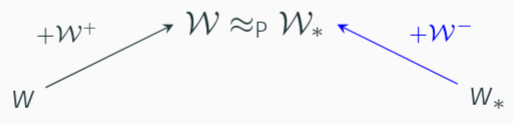This is not a word transformation as above, since it lacks the same notion of direction from $w$ to $w_*$. To remedy this, rather than considering the words in $\mathcal{W}^-$ as being added to $w_*$, we consider them subtracted from $w$ (hence the naming convention):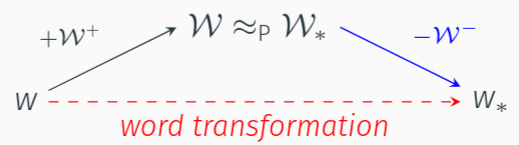Where added words narrow context, subtracted words can be thought of as broadening the context.

We say there exists a word transformation from word $w$ to word $w_*$, with transformation parameters $\mathcal{W}^+$, $\mathcal{W}^-\subseteq\mathcal{E}$ iff $\ \{w\}\!\cup\!\mathcal{W}^+ \approx_\text{P} \{w_*\}\!\cup\!\mathcal{W}^-$.

#### Intuition

The intuition behind word transformations mirrors simple algebra, e.g. 8 is made equivalent to 5 by adding 3 to the right, or subtracting 3 from the left. Analogously, with paraphrasing as a measure of equivalence, we can identify words ($\mathcal{W}^+, \mathcal{W}^-$) that when added to/subtracted from $w$, make it equivalent to $w_*$. In doing so, just as 3 describes the difference between 8 and 5 in the numeric example, we find words that describe the difference between $w$ and $w_*$, or rather, how “$w$ is to $w_*$”.

With our initial paraphrases, words could only be added to $w$ to describe its semantic difference to $w_*$, limiting the tools available to discrete words in $\mathcal{E}$. Now, differences between other words can also be used offering a far richer toolkit, e.g. the difference between $man$ and $king$ can crudely be explained by, say, $royal$ or $crown$, but can more closely be described by the difference between $woman$ and $queen$.

### Interpreting Analogies

We can now mathematically interpret the language of an analogy:

We say ”$w_a$ is to $w_{a^*}$ as $w_b$ is to $w_{b^*}\!$” iff there exist $\mathcal{W}^+\!, \mathcal{W}^-\!\subseteq\!\mathcal{E}$ that serve as transformation parameters to transform both $w_a$ to $w_{a^*}$ and $w_b$ to $w_{b^*}$.

That is, within the analogy wording, each instance of “is to” refers to the parameters of a word transformation and “as” implies their equality. Thus the semantic differences within each word pair, as captured by the transformation parameters, are the same – fitting intuition and now defined explicitly.

So, an analogy is a pair of word transformations with common parameters $\mathcal{W}^+, \mathcal{W}^-$, but what are those parameters? Fortunately, we need not search or guess. We show in the paper (Sec 6.4) that if an analogy holds, then any parameters that transform one word pair, e.g. $w_a$ to $w_{a^*}$, must also transform the other pair. As such, we can chose $\mathcal{W}^+\!=\!\{w_{a^*}\!\}$, $\mathcal{W}^-\!=\!\{w_{a}\}$, which perfectly transform $w_a$ to $w_{a^*}$ since $\{w_a, w_{a^*}\!\}$ paraphrases $\{w_{a^*\!}, w_a\}$ exactly (note that ordering is irrelevant in paraphrases, e.g. $\{man$, $royal\}$ paraphrases $\{royal$, $man\}$). But, if the analogy holds, those same parameters must also transform $w_b$ to $w_{b^*}$, meaning that $\{w_b, w_{a^*}\}$ paraphrases $\{w_{b^*}, w_a\}$.

 Thus, $\!`w_a$ is to $w_{a^*}$ as $w_b$ is to $w_{b^*}\!\!"$ if and only if $\{w_b, w_{a^*}\}$ paraphrases $\{w_{b^*}, w_a\}$.

This completes the chain:

• analogies are equivalent to word transformations with common transformation parameters that describe the common semantic difference;
• those word transformations are equivalent to paraphrases, the first of which is rendered trivial under a particular choice of transformation parameters;
• the second paraphrase leads to a geometric relationship between PMI vectors \eqref{eq:two}, subject to the accuracy of the paraphrase ($\rho$) and dependence error terms ($\sigma, \tau$); and
• under low-dimensional projection (induced by the loss function), the same geometric relationship manifests in word embeddings of analogies \eqref{eq:one}, as seen in word embeddings of W2V and Glove.

Returning to an earlier plot, we can now explain the “gap” in terms of paraphrase ($\rho$) and dependence $(\sigma, \tau$) error terms, and understand why it is small, often smallest, for the word completing the analogy.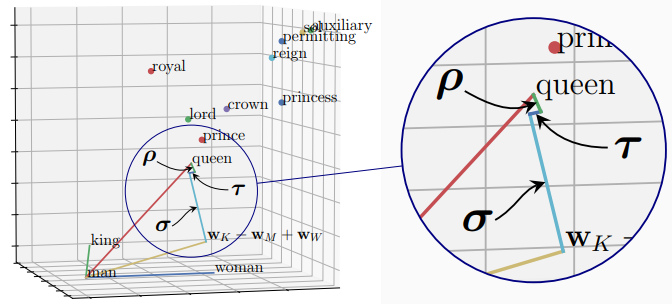Several other works aim to theoretically explain the analogy phenomenon, in particular:

• Arora et al. (2016) propose a latent variable model for text generation that is claimed inter alia to explain analogies, however strong a priori assumptions are made about the arrangement of word vectors that we do not require. More recently, we have shown that certain results of this work contradict the relationship between W2V embeddings and PMI (Levy & Goldberg).
• Gittens et al. (2017) introduce the idea of paraphrasing to explain analogies, from which we draw inspiration, but they include several assumptions that fail in practice, in particular that word frequencies follow a uniform distribution rather than their actual, highly non-uniform Zipf distribution.
• Ethayarajh et al. (2019) look to show that word embeddings of analogies form parallelograms by considering the latter’s geometric properties. However, there are several issues: (i) that all points must be co-planar is assumed without explanation; (ii) that opposite sides must have similar direction is omitted (as such, a “bow-tie” shape satisfies their Lemma 1); and (iii) that opposite sides must have similar Euclidean distance is translated to a statistical relationship termed “csPMI” – unfortunately that translation is erroneous since it relies on embedding matrix $\mathbf{W}$ being a scalar multiple ($\lambda$) of $\mathbf{C}$, which is false and, further, csPMI bears no connection to analogies or semantics.

Our work therefore provides the first end-to-end explanation for the geometric relationship between word embeddings observed for analogies.

## Further Work

Two recent works build on this paper:

• “What the Vec? Towards Probabilistically Grounded Embeddings” extends the principles of this work to show how W2V and Glove (approximately) capture other relationships such as relatedness and similarity, what certain embedding interactions correspond to and, in doing so, how the semantic relationships of relatedness, similarity, paraphrasing and analogies mathematically inter-relate.
• “Multi-relational Poincaré Graph Embeddings” [code] draws a comparison between analogies and relations in Knowledge Graphs to develop state-of-the-art representation models in both Euclidean and Hyperbolic space.